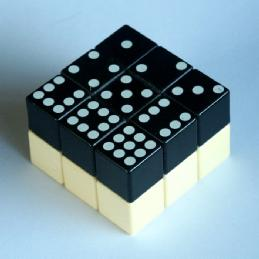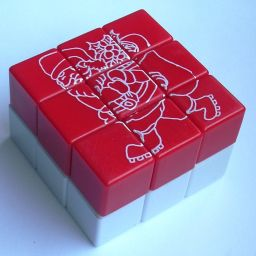# Rubik's DominoThis is like a 2x3x3 Rubik's cube. The sides which are 2×3 can obviously only do half turns. The top and bottom layer have different colours, and the pieces are simply numbered 1-9 with dots.

In the solved position, the top layer is simply numbered from left to right, from top to bottom using the numbers 1 to 9. The bottom layer pieces can be solved in two ways. You could give it the same pattern as the top layer, of it could be done in mirror image (right to left, top to bottom). In the latter case, each numbered piece in the top layer should have the same number as the piece directly underneath it. On many dominos it seems that the mirror solution is the intended one, as the number seven corner pieces are reflections of each other.

There were mostly two different mechanisms used in the original dominos. The most common use the split-axle mechanism, in which there is a core with four long axles for the sides and which is cut into upper and lower halves for the two layers. These dominos usually have mirror-image sevens on the outside. Rarer is the groove mechanism, as these tend to fall apart when they become worn out. These tend to have identical sevens on the outside.

The Magic Domino was patented by Ernő Rubik on 29 March 1983, US 4,378,116.

Note that the orientation of the centre pieces do not matter, as the number 5 is represented by a symmetric arrangement of dots. Some rare dominos have pictorial designs on them, and then the orientation of the centres becomes visible.

If your browser supports JavaScript, then you can play the Domino by clicking the link below:

## The number of positions:

There are 8 corners and 8 edges, giving a maximum of 8!·8! positions. This limit is not reached because the orientation of the puzzle does not matter. There are 4 equivalent ways to orient the puzzle with a white centre on top, so this leaves 8!·8!/4 = 406,425,600 distinct positions.
If the centre orientation is visible, then there seem to be 4·4 possible orientations of the two centres. There is a parity constraint however, as the parity of the number of quarter turns of the centres must be equal to the parity of the corner permutation. This means that the centre orientations only increase the number of positions by a factor of 8, giving 8!·8!·8/4 = 3,251,404,800 distinct positions.

I have used a computer to calculate God's algorithm for the Domino, and the results in the table below show that any position can be solved in at most 18 moves (13.290 on average), or 19 (14.156 on average) if every a half turn of the U or D layer counts as two moves.

Click to show full table.
DepthHTMQTM
011
176
23427
3168120
4807529
53,7682,280
617,2509,797
777,69641,602
8342,305172,877
91,472,169703,124
106,052,4972,753,752
1122,364,5389,959,476
1265,693,10931,168,445
13121,339,53774,084,200
14127,175,987115,192,427
1558,226,238108,804,334
163,627,11753,758,865
1732,3449,617,394
1828156,080
19264
Total   406,425,600   406,425,600

## Notation:

Let the 4 sides be denoted by L, R, F, B (left, right, front, back), and the top and bottom by U and D (up, down). Clockwise quarter turns of the top and bottom layer are denoted just by the letter U or D, half turns are denoted by U2 and D2, and anti-clockwise turns by U' and D'. A turn of one of the sides is simply a single letter. Sometimes a whole sequence in brackets is followed by a 2 or 3, indicating that the sequence as a whole is to be repeated two or three times.

## Solution 1:

Phase 1: Solve the top layer corners.

1. UFL - If not in the U layer, turn a side to get it there and hold the domino so that it is in the correct corner.
2. UFR - If in U layer but not correct then do B to put it in D layer. Rotate D until it is at DBR and then do R.
3. UBR - If in U layer but not correct then do B to put it in D layer. Rotate D until it is at DBL and then do B.
4. UBL - If not correct then rotate D until it is at DBL and do BDBD'B.

Phase 2: Solve the bottom layer corners.

1. Use one of the following:
1. Swap DBL and DBR. Do RF (D'FDR)2 D'.
2. Swap DFL and DBR. Do (FD2RD)2 RD2FD'.

Phase 3: Move the edge pieces to their correct layer.

1. Turn U/D to get a position where you can use one of the following:
1. Swap DL-UR. Do R (D2F)3 R.
2. Swap UF-DF and UR-DR. Do (FR)3.
3. Swap UF-DF and UB-DB. Do FRLBRL.

Phase 4: Solve the edge pieces of the bottom layer.

1. Rotate the whole domino to get to a position where you can use one of the following:
1. Swap DF-DR. Do: FU' FU2 RF U2 FR U'F.
2. Swap DF-DB. Do: (R D2)3.
3. Swap DF-DB, DL-DR. Do: FRLB U FRLB D'.
4. Swap DF-DR, DL-DB. Do: FBD F RL B RL D'BF.
5. Cycle DL->DF->DR->DL. Do: FD'(F R D2 R)2 DF.
6. Cycle DL->DB->DR->DF->DL. Do: RL D FB U F RL BL D2R D2R.
7. Cycle DL->DF->DB->DR->DL. Do: RD FB DFD' FB D'RD2 .

Phase 5: Solve the top layer edges.

1. Turn over the domino.
2. Repeat phase 4.

Phase 6: Orient the centres. (Dominos with picture designs only)

1. If both centres need a quarter turn, then do (R F U2 F R U2 L B) U (B L U2 R F U2 F R) D'
2. If the top centre needs a half turn, then do (R F U2 R F R U2 R F U)2
3. Turn over the puzzle and do step b for the other centre. If this final centre needs a quarter turn, then either the puzzle has been taken apart and reassembled incorrectly, or there are two identical corners that you will need to swap. In the latter case, go back to phase 2 to swap them and solve the rest again.

This solution uses M to denote a turn of the middle layer that lies between the R and L faces. Furthermore, a small letter r denotes turning the R face together with the middle slice, i.e. it is just MR.

## Solution 2:

This fast solution was devised by Stefan Pochmann.

Phase 1: Solve bottom layer edges.

1. If there is an edge in the top layer that belongs in the bottom layer, then
1. Turn the top layer so that the piece lies at the correct side, directly above where it belongs.
Note that 2 belongs in the B face, 4 in the L face, 6 in the R, 8 in the F face.
2. Turn that side face, putting the edge piece into position.
2. Repeat step a as often as you can.
3. If not yet all bottom layer edges are correctly solved, turn a side face to bring any incorrect bottom layer edge to the top, and go back to step a.

Phase 2: Solve the bottom layer corners.

1. If there is a corner in the top layer that belongs in the bottom layer, then
1. Turn the top layer so that the corner lies directly above where it belongs.
2. Put the corner in place using one of the move sequences below:
1 at the BL corner: L U L U' L
3 at the BR corner: R U' R U R
7 at the FL corner: L U' L U L
9 at the FR corner: R U R U' R
2. Repeat step a as often as you can.
3. If not yet all bottom layers are correctly solved, you could use one of the sequences from step a to displace one of the incorrect bottom layer corners, and then do step a proper to solve it.
Alternatively, you could use one of the following sequences to swap two corners:
1. Swap diagonally FR, BL corners: (L U') (R U R U' R U) L
2. Swap adjacent FR, FL corners: (R U2) [U'D] (R U R U' R) [UD'] U2 R

Phase 3: Solve top layer corners.

1. Turn U to put as many corner pieces correct as possible.
2. If two corners need to be swapped, then turn U so that one of the corners is at FR, the other at the BL or BR. Then do one of the following:
1. Swap adjacent FR, BR corners: (R U R U' R) [U'D] (R U' R U R U') [UD']
2. Swap diagonal FR, BL corners: (R U') (R U' R U R D' R U R U' R D R)
Then turn U to put the corners in position again.

Phase 4: Solve the edge pieces of the top layer.

1. Examine the edges to see how they should be moved to put them in their correct places. Turn the whole puzzle to hold it in a position where one of the following sequences will solve it:
1. F->B->L anti-clockwise 3-cycle: rU (R D R U)2 rD
2. B->F->L clockwise 3-cycle: rU' (R D' R U')2 rD'
3. F-B, L-R opposite swaps: M U M U2 M U M
4. B-R, F-L adjacent swaps: M U M F M F U' M
5. F-R adjacent swap: (R U)2 (R U2)2 R U R U' R
6. F-B opposite swap: (R U2)3
7. F->R->B->L anti-clockwise cycle: (R U r U r) (R U R U R) (r U r U R)
8. F->L->B->R clockwise 4-cycle: (R U' r U' r) (R U' R U' R) (r U' r U' R)
9. F->B -> R->L zig-zag 4-cycle: (U r U r) (R U R U' R) (r U' r U)

## Nice sequences:

1. Mirror both faces: R U (RL F)2 U F L U2 R F U2 F
2. Mirror top face: R F U' F U2 R (U F)2 U2 R U' F R
3. Turn top centre clockwise, bottom centre anti-clockwise: (R F U2 F R U2 L B) U (B L U2 R F U2 F R) D'
4. Turn both centres a half turn: (R B R L F R) U2 (R B R L F R) D2
5. Turn the top centres a half turn: (R F U2 R F R U2 R F U)2